GPT，也就是 Transformer Decoder 结构做文本生成时有一个致命问题。先来看看 Encoder 推理是怎么做的，每个 timestep 都能看到所有 timestep ，推理时所有 timestep 一层层向后计算，一把过。于是内存相关开销就是$O(N)$ , 而计算相关开销就是$O(N^2)$ ，其中 N 为序列长度。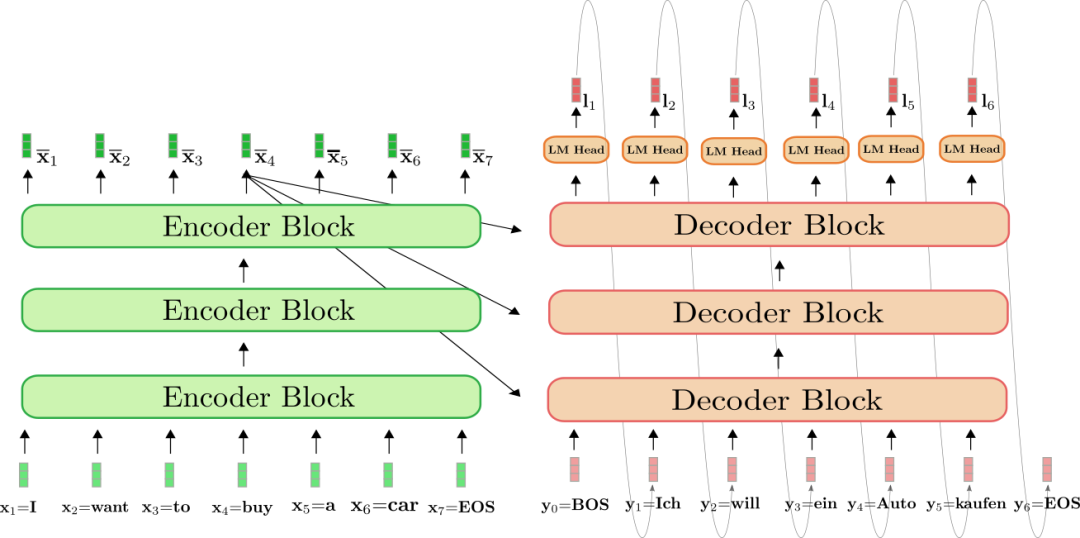### KV Cache

Decoder 每次前向，当前 timestep 计算 Attention 要用到的部分，如之前 timestep 的 KV （Key 和 Value）值都计算过的，只是之前每次前向完后给计算结果都丢掉，只保留最后输出。

SRAM是静态随机存储器，速度非常快，但成本较高。DRAM是动态随机存储器，成本较低，但速度比SRAM慢

### 冯诺依曼架构和 Memory Wall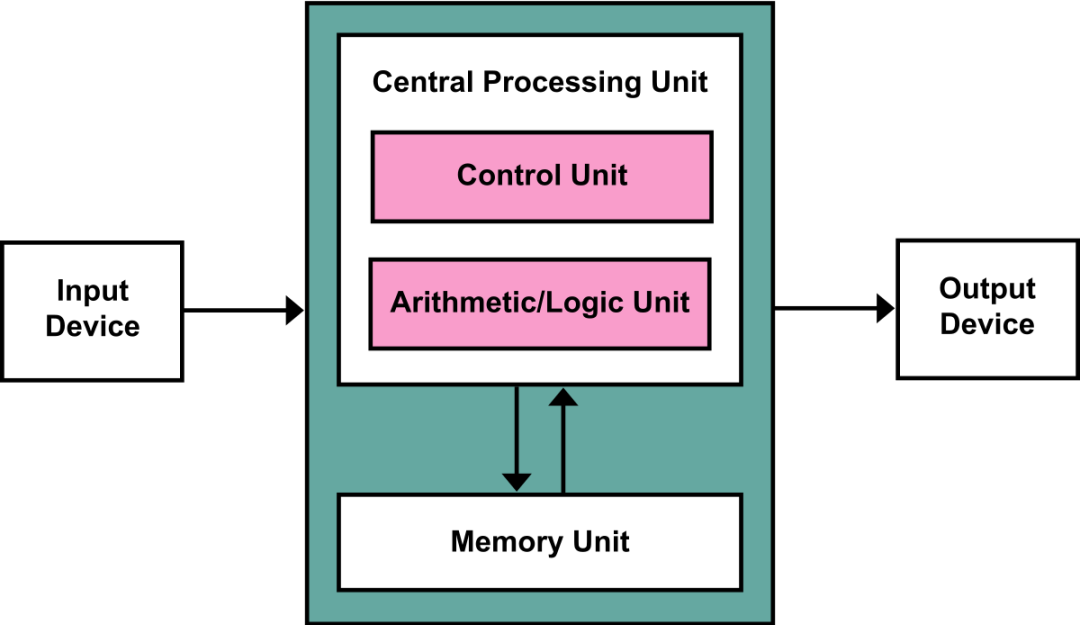Flash Attention算法背后的主要思想是分割输入，将它们从慢速HBM加载到快速SRAM，然后计算这些块的 attention 输出。在将每个块的输出相加之前，将其按正确的归一化因子进行缩放，从而得到正确的结果。

vLLM 主要用于快速 LLM 推理和服务，其核心是Paged Attention同样，MQA 也是一个软件层面上翻墙的一个方法。这是一种受操作系统中虚拟内存和分页经典思想启发的注意力算法。与传统的注意力算法不同，Paged Attention 允许在非连续的内存空间中存储连续的 key 和 value 。具体来说，Paged Attention 将每个序列的 KV cache 划分为块，每个块包含固定数量 token 的键和值。在注意力计算期间，Paged Attention 内核可以有效地识别和获取这些块。

### MHA 到 MQA 到 GQA

MQA 的方法很简单，难的是看到这样的方法后，能立刻想到它为什么好。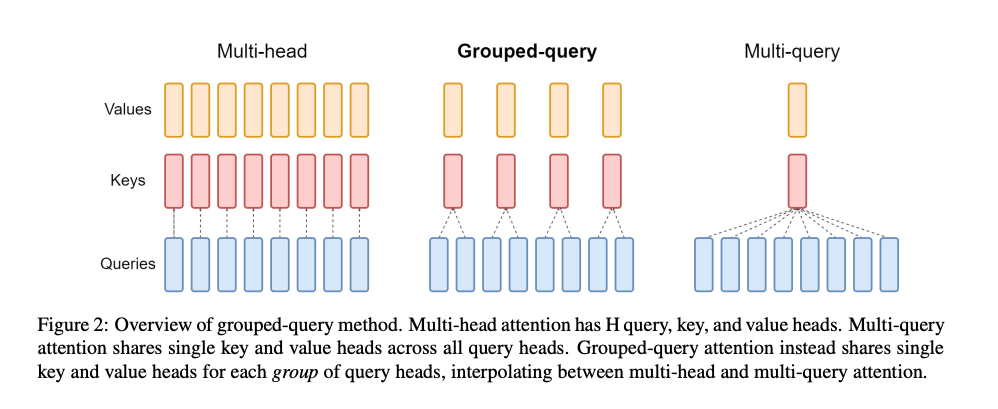MQA（Multi-Query Attention） 则是，让 Q 仍然保持原来的头数，但 K 和 V 只有一个头，相当于所有的 Q 头共享一组 K 和 V 头，所以叫做 Multi-Query 了。实现改变了会不会影响效果呢？确实会影响但相对它能带来的收益，性能的些微降低是可以接受的。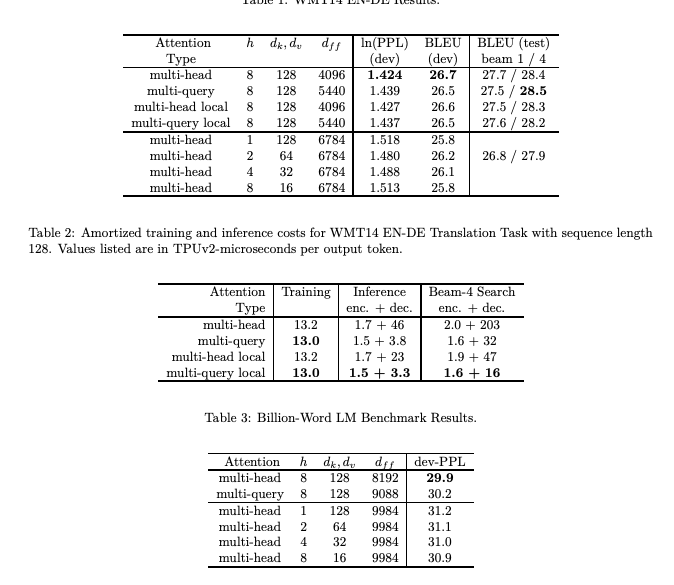GQA(Grouped-Query Attention) 呢，是 MHA 和 MQA 的折衷方案，既不想损失性能太多，又想获得 MQA 带来的推理加速好处。具体思想是，不是所有 Q 头共享一组 KV，而是分组一定头数 Q 共享一组 KV，比如上面图片就是两组 Q 共享一组 KV。

LLAMA2 中给出了效果对比，可以看到相比起 MQA，GQA的指标看起来还是要好些的。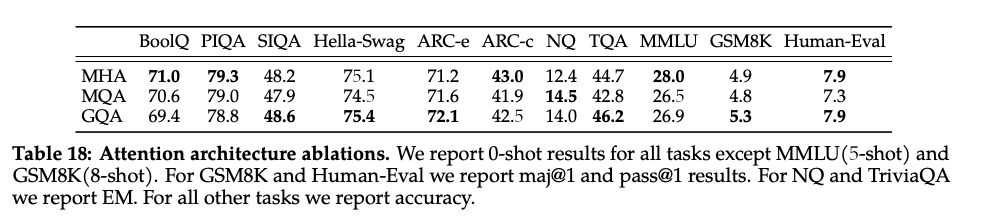MQA 和 GQA 形式在推理加速方面，主要是通过两方面来完成：

• 降低了从内存中读取的数据量，所以也就减少了计算单元等待时间，提高了计算利用率；
• KV cache 变小了 head_num 倍，也就是显存中需要保存的 tensor 变小了，空出来空间就可以加大 batch size，从而又能提高利用率。

### MQA 的推导

$3(h a d+b a)+4(b h n d+b h d) \Rightarrow 3\left(a^2+b a\right)+4(b n a+b a) \Rightarrow 3 a^2+4 b n a+7 b a \Rightarrow O\left(b n a+a^2\right)$

$3 b a^2+2 b h n d \Rightarrow 3 b a^2+2 b a n$

$\frac{M}{A}=O\left(\frac{b n a+a^2}{b a^2}\right)=O\left(\frac{n}{a}+\frac{1}{b}\right)$

$(h a d+b a)+2(a d+b a)+2(b n d+b d)+(b h d+b n d)+b h n+(b h n+b n d) \Rightarrow a^2+4 b a+2 a d+2 b d+4 b n d+2 b h n \Rightarrow O\left(b a+a^2+b n d+b h n\right)$

$\frac{M}{A}=O\left(\frac{b a+a^2+b n d+b h n}{b a^2}\right)=O\left(\frac{1}{a}+\frac{1}{b}+\frac{n}{a h}+\frac{n}{a d}\right)$